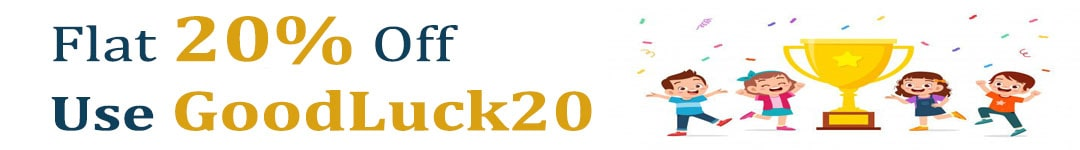International Maths Olympiad Forum By SOF Olympiad Trainer - Page 607

# User ForumSubject :IMO    Class : Class 6

## Ans 1:

Class : Class 6
5/24 x=3/8X=3/8-5/24X=4/24So 24 out of 4 rectangular must be shaded

## Ans 2:

Class : Class 6

Subject :IMO    Class : Class 6

## Ans 1:

Class : Class 9
A=Twice of B ,C=65,so as per question, A=C+17 ,then A will be 65 +17=82 then B will be half of A that is 41

Subject :IMO    Class : Class 3

Class : Class 6
U

## Ans 2:

Class : Class 10
To tip the scale to the right, the weight of the chocolates should be more than 37
Weight of 1 chocolate = 4 gms
Weight of 9 chocolates = 36 gms
Weight of 10 chocolates = 40 gms
Hence atleast 10 chocolates are needed to tip the scale to the right.

## Ans 3:

Class : Class 6
the answer should be 10

Subject :IMO    Class : Class 4

Class : Class 5

## Ans 2:

Class : Class 10
6923
1. '6' is double of '3'
2. '9' is third multiple of 3 and '3' is 1/3 of '9'
3. '2' is the smallest even number

Subject :IMO    Class : Class 5

## Ans 1:

Class : Class 6
b is even number but all other 3 are odd numbers so ans should be b

## Ans 2:

Class : Class 4
it can be option B but there is an other formula. Add all the digits of all the 4 options. Option A,B,C would be 27 but option D would be 26. That makes the difference between option A,B,C with D. So the answer is option D.

## Ans 3:

Class : Class 10
c and d as solution is given of adding digitsâºð¤

Class : Class 5

## Ans 5:

Class : Class 6
B

Subject :IMO    Class : Class 6

Class : Class 6

## Ans 2:

Class : Class 10

Corrected the question. Minus sign was missing

Solution:
David's current age = 3x -2
David's age eight years before = 3x -2 -8 = 3x - 10
Sally's age = David's age eight years before = 3x -10
Sally's age after 4 years = 3x - 10 + 4 = 3x -6

Subject :IMO    Class : Class 5

## Ans 1:

Class : Class 9
66 plus 82 is 148 and only 120 bags are there so 148 minus 120 , 28 red haversacks will be there

Subject :IMO    Class : Class 10

## Ans 1:

Class : Class 10
5%

Subject :IMO    Class : Class 6

## Ans 1:

Class : Class 8
the sum of the square of every numbers are the numbers given at the below

Subject :IMO    Class : Class 4

## Ans 1:

Class : Class 8
Answer is B Saturday

## Ans 2:

Class : Class 4
Answer is A Thursday

Class : Class 7
The answer is B

## Ans 4:

Class : Class 5
Answer is b saturday

Class : Class 4

Class : Class 5

Class : Class 4
super dveep jain

## Ans 8:

Class : Class 4
answer is b saturday

## Ans 9:

Class : Class 5
yeah answer is b saturdaybecause 1 month from19 december is 18 jan